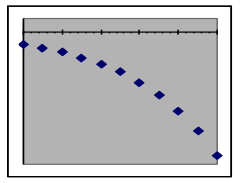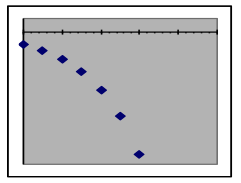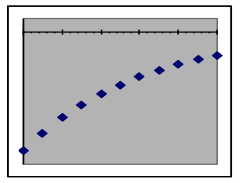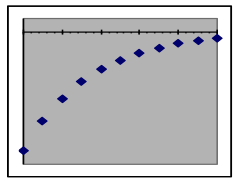## J1.05: Section 1 Part 4

Negative output values in exponential models: When the initial amount is a negative number (this usually happens when the y value describes a difference from a reference value), the only difference is that all of the model’s output values will be negative and the graphs will thus be reflected around the x-axis, “upside down” compared to models with positive initial values.

 Relationship of exponential models with negative initial amounts to the x-axisExponential growth Faster growth rate Exponential decay Faster decay rate

Example 5: The table to the right shows the temperature of a soda can as it warms up in a room whose temperature is 75°F.   Use an exponential model to: [a] estimate the decay rate per minute of the difference between the can temperature and the room temperature, [b] predict the can temperature at 20 minutes, and [c] estimate the half-life of the warming process.

Solution:

Since the temperature of the can is not converging on zero, we can’t directly use can temperature as a variable in an exponential-decay model. Instead, we will subtract the room temperature from the can temperature and use that difference as the output variable for the model.

Put this dataset into the exponential-model spreadsheet, then adjust the parameters to make the model fit the data. You will find that the data is well matched by an exponential model whose initial-value parameter is -40 and whose “growth” rate is -0.09 (which is really a decay rate since it is negative).

Original data

 Minutes out of cooler Temperature of can 0 35 5 50 10 59 15 65

Redefined data

 Minutes out of cooler Degrees from 75° F 0 -40 5 -25 10 -16 15 -10

Evaluating the model for x = 20 gives a predicted difference at that time of 6.1 degrees below the room temperature of 75°F.

The data point whose y value is furthest from zero is the first one at (x=0, y=-40). The line halfway to the x-axis is at y=–20, which crosses the model graph at between 7 and 8 minutes.